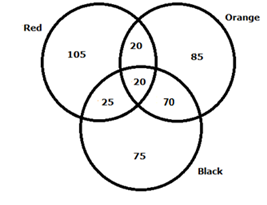# SBI Clerk Prelims 2021 Quantitative Aptitude Questions (Day-10)

Dear Aspirants, Our IBPS Guide team is providing new series of Quantitative Aptitude Questions for SBI Clerk Prelims 2020 so the aspirants can practice it on a daily basis. These questions are framed by our skilled experts after understanding your needs thoroughly. Aspirants can practice these new series questions daily to familiarize with the exact exam pattern and make your preparation effective.

Start Quiz

Caselet

Directions (01 – 05): Study the following information carefully and answer the questions given below.

There are 400 students in the class, each of them like at least one of the three different colors Red, Black and Orange. 25 students like both Black and Red but not Orange. Out of 26.25% of the students like only Red, 21.25% of the students like only Orange and three – sixteenth of the students who like only Black. 20 students like all the three colors together. Ratio of the number of students who like both Red and Orange but not Black to the number of students who likes all the colors together is 1: 1.

1) What is the difference between the number of students who like Red and number of students who like Black?

A.20

B.25

C.15

D.10

E.None of these

2) What is the ratio of the number of students like at least 2 colors to the number of students like at most one color?

A.10: 23

B.20: 63

C.27: 53

D.20: 67

E.None of these

3) Find the number of students like exactly two colors?

A.115

B.125

C.110

D.130

E.None of these

4) The number of students like both Orange and Black but not Red is approximately what percent of the total number of students like only Red?

A.76.5%

B.66.67%

C.81.5%

D.63.5%

E.59.5%

5) What is the ratio of the number of students like both Red and Black but not orange to the number of students like only Orange?

A.5: 17

B.6: 19

C.4: 15

D.3: 11

E.None of these

Simplification

Directions (06 – 10): What value should come in the place of (?) in the following questions?

6) 17*15 +? – 33(1/3) % * 729 = 81*11

A.1331

B.1728

C.1111

D.1122

E.None of these

7) 2 1/3 + 3 2/6 – 4 1/9 + 11 1/9 =?

A.12 2/3

B.10 1/3

C.12 1/3

D.10 1/9

E.None of these

8) 14.5 * 5.5 ÷ 11 – 303 ÷ 6 + 725 =?

A.960.75

B.681.75

C.1024.50

D.675.75

E.None of these

9)
16% of 18% of 3600 + 34.64 * 101.5 ÷ 203 = (?)2

A.21

B.31

C.9

D.11

E.None of these

10)  [72 *(25)2 ÷ 44*22] ÷ 36 = (?)2

A.21

B.25

C.35

D.31

E.None of these

Directions (1-5) :

Number of students likes only Red = 26.25/100 * 400 = 105

Number of students likes only Orange = 21.25/100 * 400 = 85

Number of students likes only Black = 3/16 * 400 = 75

Number of students who likes all the three colors = 20

Number of students likes both Black and Red but not Orange = 25

Number of students likes both Red and Orange but not Black = 1/1 * 20 = 20

Number of students like both Orange and Black but not Red

= 400 – (105 + 85 + 75 + 25 + 20 + 20)

= 400 – 330 = 70Required difference = (105 + 20 + 20 + 25) – (20 + 70 + 25 + 75)

= 190 – 170

= 20

Number of students like at least 2 colors = 20 + 25 + 70 + 20 = 135

Number of students like at most one color = 105 + 85 + 75 = 265

Required ratio = 135: 265

= 27: 53

Number of students like exactly two colors = 70 + 25 + 20 = 115

Required percentage = 70/105 * 100 = 66.67%

Difference = 25: 85 = 5: 17

17*15 +? – 33(1/3) % * 729 = 81*11

? = 81*11 + 1/3 * 729 – 17*15

= 891 + 243 – 255

= 879

2 1/3 + 3 2/6 – 4 1/9 + 11 1/9 =?

(2 + 3 – 4 + 11) + (1/3 + 2/6 – 1/9 + 1/9) =?

12 4/6 =?

12 2/3 =?

14.5 * 5.5 ÷ 11 – 303 ÷ 6 + 725 =?

= 14.5 * 0.5 – 50.5 +725

= 681.75

16% of 18% of 3600 + 34.64 * 101.5 ÷ 203 = (?)2

(0.16 * 0.18 *3600) + 34.64 * (101.5 ÷ 203) = (?)2

103.68 + (34.64) * 0.5 = (?)2

103.68 + 17.32 = (?)2

121 = (?)2

? = 11

[72 *(25)2 ÷ 44*22] ÷ 36 = (?)2

(72 * 625/44 * 22)/36 = (?)2

625 = (?)2

25 =?

 Check Here to View SBI Clerk Prelims 2021 Quantitative Aptitude Questions Day – 09 Day – 08 Day – 07